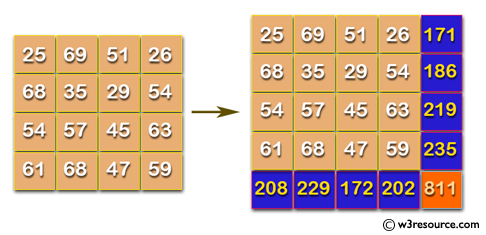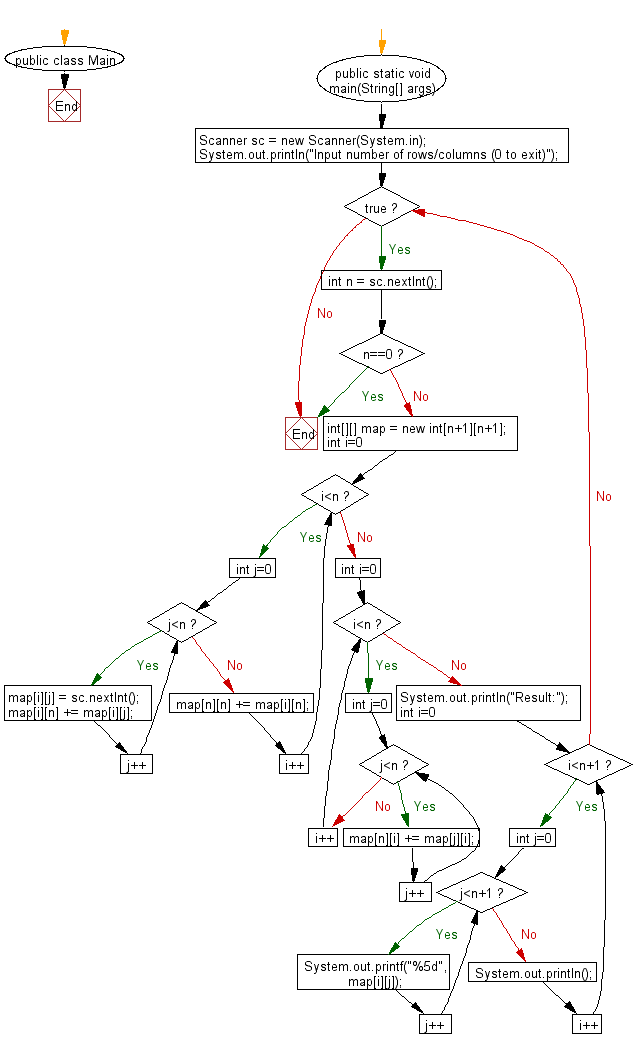# Java Exercises: Adds up columns and rows of given table as shown in the specified figure

## Java Basic: Exercise-242 with Solution

Write a Java program which adds up columns and rows of given table as shown in the specified figure:

Pictorial Presentation:Input:
n (the size of row and column of the given table)
1st row of the table
2nd row of the table
:
:
n th row of the table
The input ends with a line consisting of a single 0.
Output:
For each dataset, print the table with sum of rows and columns.

Sample Solution:

Java Code:

`````` import java.util.*;

public class Main {
public static void main(String[] args) {
Scanner sc = new Scanner(System.in);
System.out.println("Input number of rows/columns (0 to exit)");
while(true){
int n = sc.nextInt();
if(n==0)break;
int[][] map = new int[n+1][n+1];

for(int i=0;i<n;i++){
for(int j=0;j<n;j++){
map[i][j] = sc.nextInt();
map[i][n] += map[i][j];
}
map[n][n] += map[i][n];
}

for(int i=0;i<n;i++){
for(int j=0;j<n;j++){
map[n][i] += map[j][i];
}
}
System.out.println("Result:");
for(int i=0;i<n+1;i++){
for(int j=0;j<n+1;j++){
System.out.printf("%5d", map[i][j]);
}
System.out.println();
}
}
}
}
```
```

Sample Output:

```Input number of rows/columns (0 to exit)
4
25 69 51 26
68 35 29 54
54 57 45 63
61 68 47 59
Result:
25   69   51   26  171
68   35   29   54  186
54   57   45   63  219
61   68   47   59  235
208  229  172  202  811
```

Flowchart:Java Code Editor:

What is the difficulty level of this exercise?

﻿

## Java: Tips of the Day

Array vs ArrayLists:

The main difference between these two is that an Array is of fixed size so once you have created an Array you cannot change it but the ArrayList is not of fixed size. You can create instances of ArrayLists without specifying its size. So if you create such instances of an ArrayList without specifying its size Java will create an instance of an ArrayList of default size.

Once an ArrayList is full it re-sizes itself. In fact, an ArrayList is internally supported by an array. So when an ArrayList is resized it will slow down its performance a bit as the contents of the old Array must be copied to a new Array.

At the same time, it's compulsory to specify the size of an Array directly or indirectly while creating it. And also Arrays can store both primitives and objects while ArrayLists only can store objects.

Ref: https://bit.ly/3o8L2KH